×#### Thank you for registering.

One of our academic counsellors will contact you within 1 working day.

Click to Chat

1800-1023-196

+91-120-4616500

CART 0

• 0

MY CART (5)

Use Coupon: CART20 and get 20% off on all online Study Material

ITEM
DETAILS
MRP
DISCOUNT
FINAL PRICE
Total Price: Rs.

There are no items in this cart.
Continue Shopping• Complete JEE Main/Advanced Course and Test Series
• OFFERED PRICE: Rs. 15,900
• View Details

```Data Handling II (Graphical Representation of Data as Histograms) Exercise 24.1

Question: 1

Given below is the frequency distribution of the heights of 50 students of a class:

Class interval:
140−145
145−150
150−155
155−160
160−165

Frequency:
8
12
18
10
5

Draw a histogram representing the above data.

Solution:

The class limits are represented along the x-axis on a suitable scale and the frequencies are represented along the y-axis on a suitable scale. Taking class intervals as bases and the corresponding frequencies as heights, the rectangles can be constructed to obtain the histogram of the given frequency distribution as shown in the figure below: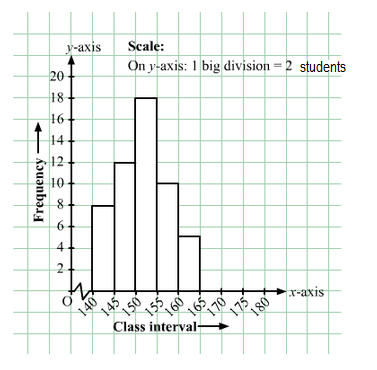Question: 2

Draw a histogram of the following data:

Class interval:
10−15
15−20
20−25
25−30
30−35
34−40

Frequency:
30
98
80
58
29
50

Solution:

The class limits are represented along the x-axis and the frequencies are represented along the y-axis on a suitable scale. Taking class intervals as bases and the corresponding frequencies as heights, the rectangles can be drawn to obtain the histogram of the given frequency distribution. The histogram is shown below: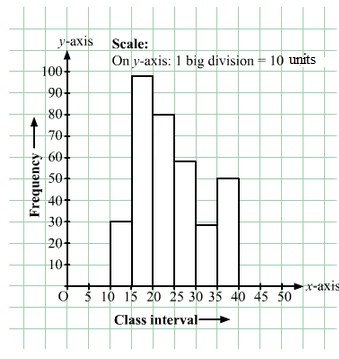Question: 3

Number of workshops organized by a school in different areas during the last five years are as follows:

Years
No. of workshops

1995−1996
25

1996−1997
30

1997−1998
42

1998−1999
50

1999−2000
65

Draw a histogram representing the above data.

Solution:

The class limits are represented along the x-axis and the frequencies are represented along the y-axis on a suitable scale. Taking class intervals as bases and the corresponding frequencies as heights, the rectangles can be constructed to obtain histogram for the given frequency. The histogram is shown below: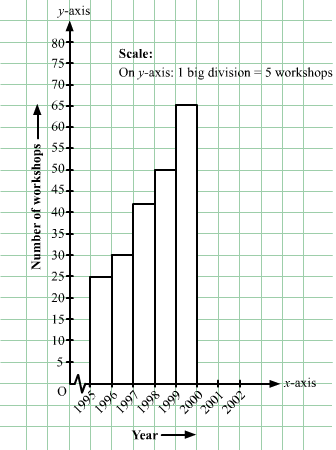Question: 4

In a hypothetical sample of 20 people the amounts of money with them were found to be as follows:
114, 108, 100, 98, 101, 109, 117, 119, 126, 131, 136, 143, 156, 169, 182, 195, 207, 219, 235, 118.
Draw the histogram of the frequency distribution (taking one of the class intervals as 50−100).

Solution:

We first prepare the frequency table for the class intervals 50−100, 100−150,..., 200−250, as shown below:

Money
Tally Amount
Frequency

50−100
98
1

100−150
114, 108, 100, 101, 109, 117, 119, 126, 131, 136, 143, 118
12

150−200
156, 169, 182, 195
4

200−250
207, 219, 235
3

The class limits are represented along the x-axis and the frequencies along the y-axis on a suitable scale. Taking the class intervals as bases and the corresponding frequencies as heights, the rectangles can be drawn to obtain the histogram of the given frequency distribution. The histogram is shown below:Question: 5

Construct a histogram for the following data:

Monthly school fee (in Rs):
30−60
60−90
90−120
120−150
150−180
180−210
210−240

Number of schools:
5
12
14
18
10
9
4

Solution:

The class limits are represented along the x-axis and the frequencies along the y-axis on a suitable scale. Taking class intervals as bases and corresponding frequencies as heights of the rectangles, the histogram of the given data can be obtained as shown in the figure below:Question: 6

Draw a histogram for the daily earnings of 30 drug stores in the following table:

Daily earnings (in Rs):
450−500
500−550
550−600
600−650
650−700

Numbers of stores:
16
10
7
3
1

Solution:

The class limits are represented along the x-axis and the frequencies along the y-axis on a suitable scale. Taking class intervals as bases and the corresponding frequencies as heights, the rectangles can be drawn to obtain the histogram of the given frequency distribution. The histogram is given below: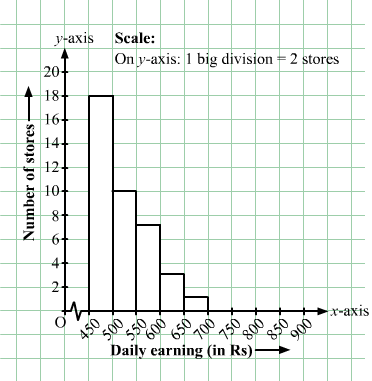Question: 7

Draw a histogram to represent the following data:

Monthly salary (in Rs)
Number of teachers

5600−5700
8

5700−5800
4

5800−5900
3

5900−6000
5

6000−6100
2

6100−6200
3

6200−6300
1

6300−6400
2

Solution:

Taking class intervals as bases and the corresponding frequencies as heights, the rectangles can be constructed to obtain the histogram of the given data. The class intervals are represented along the x-axis and the frequencies along the y-axis on a suitable scale.

The histogram representing the given data is shown below: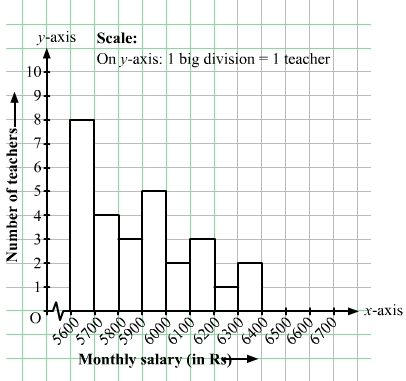Question: 8

The following histogram shows the number of literate females in the age group of 10 to 40 years in a town: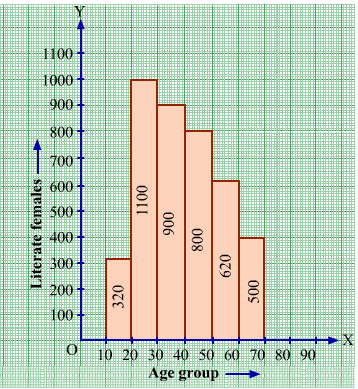(i) Write the age group in which the number of literate female is the highest.

(ii) What is the class width?

(iii) What is the lowest frequency?

(iv) What are the class marks of the classes?

(v) In which age group literate females are the least?

Solution:

(i) The highest rectangle corresponds to the highest number of literate females, which is in the interval 15−20 years.

(ii) The class intervals are 10−15, 15−20, 20−25, 30−35, 35−40. Hence, the class width is 5.

(iii) The lowest rectangle corresponds to the lowest frequency, which is 320.

(iv) The class mark is the mid-point of the class interval.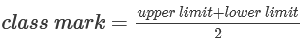Hence, the class mark of each class is as follows:

Interval
Class Mark

10−15
12.5

15−20
17.5

20−25
22.5

25−30
27.5

30−35
32.5

35−40
37.5

(v) The lowest rectangle corresponds to the least number of literate females, which is in the interval 10−15 years.

Question: 9

The following histogram shows the monthly wages (in Rs) of workers in a factory: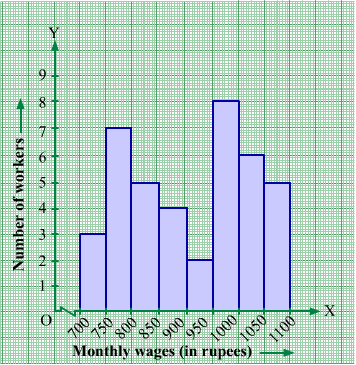(i) In which wage-group the largest number of workers are being kept? What is their number?

(ii) What wages are the least number of workers getting? What is the number of such workers?

(iii) What is the total number of workers?

(iv) What is the factory size?

Solution:

(i) In Fig 24.8, the highest rectangle corresponds to the largest number of workers. The required interval is Rs 950−1000. There are 8 workers in this interval.

(ii) The lowest rectangle corresponds to the least number of workers. The required interval is Rs 900−950. There are 2 workers in this interval.

(iii) The total number of workers is the sum of workers in all the intervals. It can be calculated as follows:

Total workers = 3 + 7 + 5 + 4 + 2 + 8 + 6 + 5 = 40 workers

(iv) The factory intervals are 750−800, 800−850, .. 1050−100. Hence, the factory size is 50.

Question: 10

Below is the histogram depicting marks obtained by 43 students of a class:

(i) Write the number of students getting the highest marks.

(ii) What is the class size?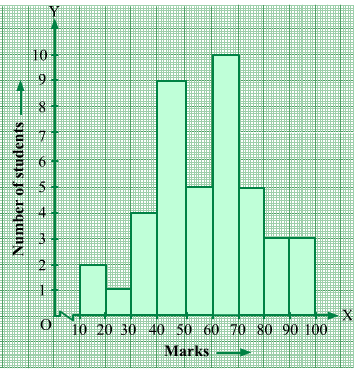Solution:

(i) In the given histogram, the interval with the highest marks is 90−100.

Three students are there in this interval because the height of the rectangle (90−100) is 3 units.

(ii) The class intervals are 10−20, 20−30, ..., 90−100. So, the class size is 10.

Question: 11

The following histogram shows the frequency distribution f the ages of 22 teachers in a school: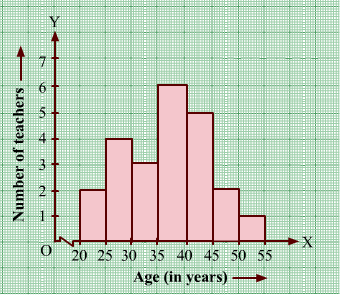(i) What is the number of eldest and youngest teachers in the school?

(ii) Which age group teachers are more in the school and which least?

(iii) What is the size of the classes?

(iv) What are the class marks of the classes?

Solution:

(i) The eldest (50−55 years) = 1 person

This is because the height of the rectangle with class interval 50−55 as base is 1 unit.

The youngest (20−25 years)= 2 persons

This is because the height of the rectangle with class interval 20−25 as base is 2 units.

(ii) The group containing the most number of teachers is 35−40 years. This is because the height of the rectangle with class interval 35−40 as base is the maximum.

The group containing the least number of teachers is 50−55 years. This is because the height of the rectangle with class interval 50−55 as base is the minimum.

(iii) Class size = The range between the upper and the lower boundaries of the class

For example, the size of the class 20−25 years is 5.

(iv)Similarly, the class marks of the other classes are 27.5; 32.5; 37.5; 42.5; 47.5; 52.5.

Question: 12

The weekly wages (in Rs.) of 30 workers in a factory are given:

830, 835, 890, 810, 835, 836, 869, 845, 898, 890, 820, 860, 832, 833, 855, 845, 804, 808, 812, 840, 885, 835, 835, 836, 878, 840, 868, 890, 806, 840

Mark a frequency table with intervals as 800-810, 810-820 and so on, using tally marks. Also, draw a histogram and answer the following questions:

(i) Which group has the maximum number of workers?

(ii) How many workers earn Rs 850 and more?

(iii) How many workers earn less than Rs 850?

Solution:

The frequency table with intervals 800−820, 810−820,...890−900 is given below:

Wage (in Rs)
Tally Wage
Frequency
Tally marks

800−810
804, 808, 806
3
III

810−820
810, 812
2
II

820−830
820
1
I

830−840
830, 835, 835, 836, 832, 833, 835, 835, 836
9
IIII IIII

840−850
845, 845, 840, 840, 840
5
IIII

850−860
855
1
I

860−870
869, 860, 868
3
III

870−880
878
1
I

880−890
885
1
I

890−900
890, 898, 890, 890
4
IIII

The class limits are represented along the x-axis and the frequencies along the y-axis on a suitable scale. Taking class intervals as bases and the corresponding frequencies as heights, the rectangles can be drawn to obtain the histogram of the given frequency distribution. The histogram is shown below: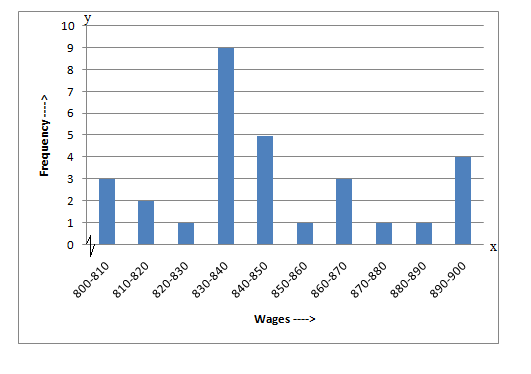(i) The group that has the maximum number of workers is represented as the highest rectangle. It is in the interval 830−840.

(ii) The number of workers who earn Rs 850 or more can be calculated from frequency table in the following manner:

1 + 3 + 1 + 1 + 4 = 101 + 3 + 1 + 1 + 4 = 10

(iii) The number of workers who earn less than Rs 850 can be calculated from frequency table in the following manner:

3 + 2 + 1 + 9 + 5 = 203 + 2 + 1 + 9 + 5 = 20
```### Course Features

• 728 Video Lectures
• Revision Notes
• Previous Year Papers
• Mind Map
• Study Planner
• NCERT Solutions
• Discussion Forum
• Test paper with Video Solution